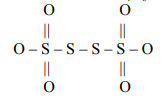A brown coloured mixture of two gases is obtained by the reduction of 6N nitric acid with metallic
copper. This mixture on cooling condenses to a blue liquid which on freezing (-30o C) gives a blue solid.
The correct choice for blue liquid or solid is.

(A) It is referred to as the anhydride of nitrous acid.
(B) It is an acidic oxide and hence dissolves in alkalies producing nitrites.
(C) It can also be prepared by the action of 50% HNO3 on arsenious oxide and then cooling to 250K.
(D) All of these.

Concept Questions :-

group 15 ,preparation and properties
High Yielding Test Series + Question Bank - NEET 2020

Difficulty Level:

Select the incorrect statement

(A) Nitrous oxide supports combustion more vigorously than air.

(B) Phosphorous pentaoxide dehydrates nitric acid forming nitrogen pentaoxide.

(C) Reactivity order of various allotropic forms of phosphorous is white > red > black.

(D) Red phosphorous changes to white phosphorus on heating in the atmosphere of $C{O}_{2}$  or coal gas at 573 K.

Concept Questions :-

group 15 ,preparation and properties
High Yielding Test Series + Question Bank - NEET 2020

Difficulty Level:

Phosphate rock (fluorapatite) on reaction with orthophosphoric acid produces:

1. $Ca{\left({H}_{2}P{O}_{4}\right)}_{2}+Ca{F}_{2}$

2. $C{a}_{3}{\left(P{O}_{4}\right)}_{2}+Ca{F}_{2}+{H}_{2}O$

3. $Ca{\left({H}_{2}P{O}_{4}\right)}_{2}+HF$

4. $C{a}_{3}{\left(P{O}_{4}\right)}_{2}+HF+{H}_{2}O$

Concept Questions :-

group 15 ,preparation and properties
High Yielding Test Series + Question Bank - NEET 2020

Difficulty Level:

$N{H}_{4}Cl{O}_{4}+HN{O}_{3}\left(dilute\right)\to HCl{O}_{4}+\left[X\right]$

$\left[X\right]\stackrel{∆}{\to }Y\left(g\right)$

$\left[X\right]$ and $\left[Y\right]$ are respectively.

(a)        (c)

(b)                 (d) None

Concept Questions :-

group 15 ,preparation and properties
High Yielding Test Series + Question Bank - NEET 2020

Difficulty Level:

One of the products of the following reaction $KCNO+{\left(NH\right)}_{4}S{O}_{4}$ is :

(A) $N{H}_{4}N{O}_{2}$                              (B) $N{H}_{4}N{O}_{3}$

(C) $N{H}_{2}-CO-N{H}_{2}$                  (D) ${N}_{2}$ and $N{O}_{2}$ both

Concept Questions :-

group 15 ,preparation and properties
High Yielding Test Series + Question Bank - NEET 2020

Difficulty Level:

Which of the following orders is correct?

(1) $Sb{H}_{3}>N{H}_{3}>As{H}_{3}>P{H}_{3}$ – Boiling Point

(2) $N{H}_{3}>P{H}_{3}>As{H}_{3}>Sb{H}_{3}$  – Thermal Stability

(3) $N{H}_{3}>P{H}_{3}>As{H}_{3}>Sb{H}_{3}$  – Basic Character

(4) $N{H}_{3}>P{H}_{3}>As{H}_{3}>Sb{H}_{3}$  – Bond Angle

(A) (1), (2) and (3) only                             (B) (2), (3) and (4) only

(C) (1), (3) and (4) only                             (D) (1), (2), (3) and (4).

Concept Questions :-

group 15 ,preparation and properties
High Yielding Test Series + Question Bank - NEET 2020

Difficulty Level:

Which of the following is correct?

(A) ${S}_{3}{O}_{9}$  – contains no S - S linkage.

(B) ${S}_{2}{O}_{6}^{2-}$   – contains – O - O linkage.

(C) ${\left(HP{O}_{3}\right)}_{3}$  – contains P – P linkage.

(D) ${S}_{2}{O}_{8}^{2-}$ - contains S – S linkage.

Concept Questions :-

group 16,preparation and properties
High Yielding Test Series + Question Bank - NEET 2020

Difficulty Level:

The oxidation states of S atom in ${S}_{4}{O}_{6}^{2-}$  from left to right respectively are:(A) + 6, 0, 0, +6                          (B) +5, +1, +1, +5

(C) +5, 0, 0, +5                           (D) +3, +1, +1, +3

Concept Questions :-

group 16,preparation and properties
High Yielding Test Series + Question Bank - NEET 2020

Difficulty Level:

One gas bleaches the coloured matter by reduction temporary while the other one by oxidation
permanently. The gases are respectively:

(A)                               (B)

(C)                              (D)

Concept Questions :-

group 16,preparation and properties
High Yielding Test Series + Question Bank - NEET 2020

Difficulty Level:

${H}_{2}S{O}_{4}$  cannot be used to prepare HI from KI as:

(A) Its reaction with KI is very slow.                   (B) It oxidizes HI to iodine.
(C) It disproportionates HI.                                (D) It reduces HI.

Concept Questions :-

group 17 ,preparation and properties